Include Top

# Two Sample Mann-Whitney Test - Exact

This tool is used to compute exact P-Values. Typically this would not be necessary unless the sample sizes were smaller (each sample N <= 10 for Mann-Whitney), but this gives continuity on the example.

1. Open Customer Data.xlsx, click Sheet 1 tab (or press F4 to activate last worksheet).
2. Click SigmaXL > Statistical Tools > Nonparametric Tests - Exact > 2 Sample Mann-Whitney - Exact. If necessary, check Use Entire Data Table, click Next.
3. With Stacked Column Format checked, select Overall Satisfaction, click Numeric Data Variable (Y) >>; select Customer Type, click Group Category (X) >>; and Ha: Not Equal To . Select Exact with the default Time Limit for Exact Computation = 60 seconds.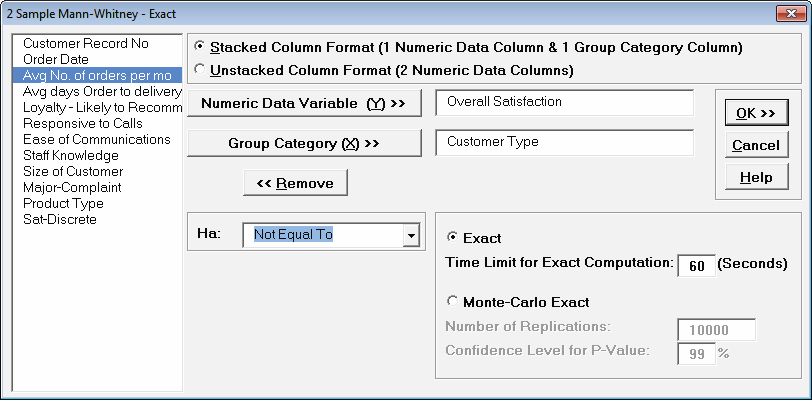Tip: If the exact computation time limit is exceeded a dialog will prompt you to use Monte Carlo or to increase the computation time. When this occurs, Monte Carlo is usually recommended. However, for this example, a slower computer may require more time than 60 seconds, so try changing the Time Limit for Exact Computation to 120 seconds.

4. Click OK. Select Customer Type 2 and 3.

5.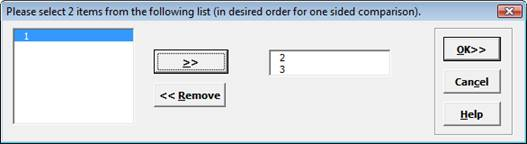6. Click OK. Resulting output:

7.Given the P-Value of .0006 we reject H0 and conclude that Median Customer Satisfaction is significantly different between Customer types 2 and 3. The Mann Whitney Statistic is identical to the above “large sample” or “asymptotic” result. The Exact P-Value is close but slightly different. This was expected because the sample size is reasonable (each sample N > 10), so the “large sample” P-Values are valid using a normal approximation for the Mann-Whitney Statistic.

The Exact P-Value was computed in seconds, but if the data set was larger, the required computation time could become excessive, and Monte Carlo would be required. We will rerun this analysis with Monte Carlo and discuss the output report.

8. Press F3 or click Recall SigmaXL Dialog to recall last dialog. Select Monte Carlo Exact with the default Number of Replications = 10000 and Confidence Level for P-Value = 99%.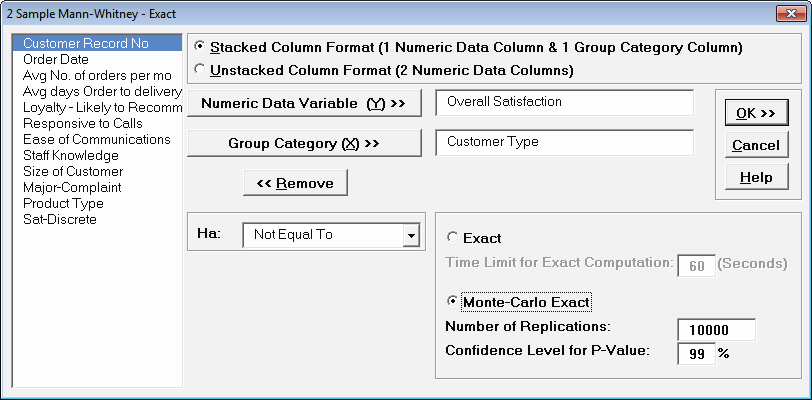Tip: 10,000 replications will result in a Monte Carlo P-Value that is typically correct to two decimal places. One million (1e6) replications will result in three decimal places of accuracy and typically require less than 60 seconds to solve for any data set.

Tip: The Monte Carlo 99% confidence interval for P-Value is not the same as a confidence interval on the test statistic due to data sampling error. The confidence level for the hypothesis test statistic is still 95%, so all reported P-Values less than .05 will be highlighted in red to indicate significance. The 99% Monte Carlo P-Value confidence interval is due to the uncertainty in Monte Carlo sampling, and it becomes smaller as the number of replications increases (irrespective of the data sample size). The Exact P-Value will lie within the stated Monte Carlo confidence interval 99% of the time.

9. Click OK. Select Customer Type 2 and 3. Click OK. Results: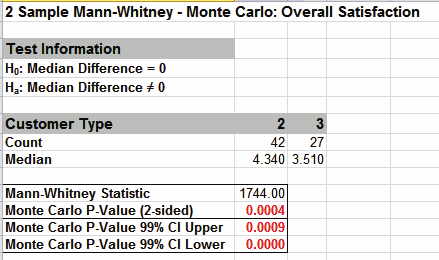The Monte Carlo P-Value here is 0.0004 with a 99% confidence interval of 0.0000 to 0.0009. This will be slightly different every time it is run (the Monte Carlo seed value is derived from the system clock). The true Exact P-Value = 0.0006 lies within this confidence interval. This was demonstrated using 10,000 replications, but with a P-Value this low, it is recommended that the number of replications be increased to 1e5 or 1e6 to get a better estimate.

10. Now we will consider a small sample problem. Open Stimulant Test.xlsx. This data is from:

Narayanan, A. and Watts, D. “Exact Methods in the NPAR1WAY Procedure,” SAS Institute Inc., Cary, NC. http://support.sas.com/rnd/app/stat/papers/exact.pdf

Researchers conducted an experiment to compare the effects of two stimulants. Thirteen randomly selected subjects received the first stimulant, and six randomly selected subjects received the second stimulant. The reaction times are in minutes. We will test the null hypothesis of no difference between the medians of the two stimulants against the alternative that stimulant 1 has smaller median reaction time than stimulant 2.

11. Select Reaction Time tab. Click SigmaXL > Statistical Tools > Nonparametric Tests – Exact > 2 Sample Mann-Whitney - Exact. If necessary, check Use Entire Data Table, click Next.
12. With Stacked Column Format checked, select Reaction Time, click Numeric Data Variable (Y) >>; select Stimulant, click Group Category (X) >>; and Ha: Less Than . Select Exact with the default Time Limit for Exact Computation = 60 seconds.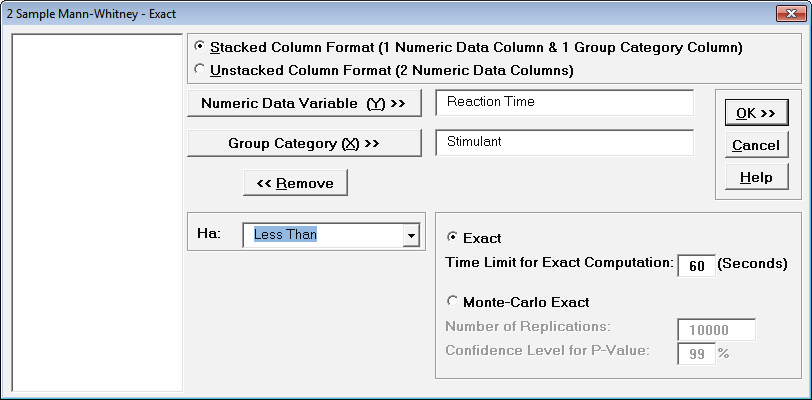13. Click OK. Select Stimulant 1 and 2.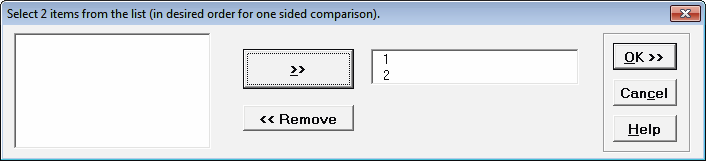This sets the order for the one sided test, so the alternative hypothesis Ha is Median 1 < Median 2.

14. Click OK. Results: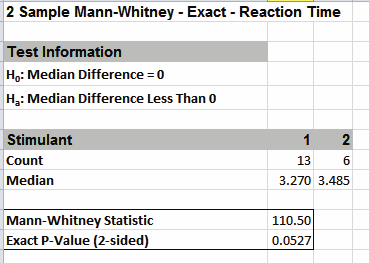With the P-Value = .0527 we fail to reject H0, so cannot conclude that there is a difference in median reaction times. This exact P-Value matches that given in the reference paper.

By way of comparison we will now rerun the analysis using the “large sample” or “asymptotic” Mann-Whitney test.

15. Select Reaction Time tab (or press F4 to activate last worksheet). Click SigmaXL > Statistical Tools > Nonparametric Tests > 2 Sample Mann-Whitney. If necessary, check Use Entire Data Table, click Next.
16. With Stacked Column Format checked, select Reaction Time, click Numeric Data Variable (Y) >>; select Stimulant, click Group Category (X) >>; and Ha: Less Than.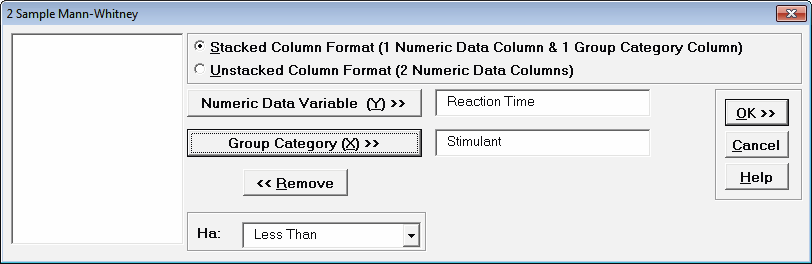17. Click OK. Select Stimulant 1 and 2. Click OK. Results:Now with the P-Value = .0421 we incorrectly reject H0.

The difference between exact and large sample P-Value is small but it was enough to lead us to falsely conclude that stimulant 1 resulted in a reduced median reaction time.

In conclusion, whenever you have a small sample size and are performing a Nonparametric test, always use the Exact option.

# Web Demos

Our CTO and Co-Founder, John Noguera, regularly hosts free Web Demos featuring SigmaXL and DiscoverSim